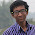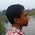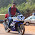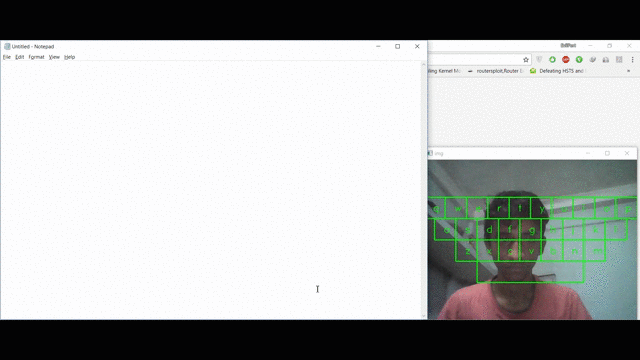## Friday, 6 October 2017

### Gesture driven Virtual Keyboard using OpenCV + Python

Hello Readers, long time no see. In this tutorial, I will be teaching you how to create a gesture driven Virtual Keyboard with OpenCV and Python. Though I am not sure if I can call this an Augmented Reality Keyboard (PLZ TELL ME IF YOU KNOW), but this is still an awesome project.

## Outcome

So before going on and blabbering about this project I would like you to see the end results. The actual video is of 1 minute and 40 seconds. It is speeded up to compress the video in 30 seconds.
You can see that the keyboard contains only 26 alphabets and a spacebar and I am wearing yellow paper on my finger with which I am trying to simulate a keyboard click.

### Requirements

1. A computer with a good camera
2. A yellow (though any other color can be used) piece of paper to be worn in a finger (for color segmentation).
3. OpenCV for Python 3
4. PyAutoGui for Python 3
5. Python 3
6. Text Editor like Sublime Text 3 or Atom
7. A little bit of knowledge in Maths

### Steps that we are going to take

1. Get the corner coordinates of each and every key that is used to design the keyboard.
2. Write a function to recognize the click.

## Let's start coding...

Before designing our project we need to first know about our constraints. Obviously we have some restrictions regarding the GUI of the keyboard that is to be designed. Let me list them:-
1. Every key needs to be of a fixed width and height
2. Square keys look much better than any other shape
3. We need to have equal margins in both sides i.e and left and right side of each row
4. The total width of a row should not exceed the width of the frame. The same goes for the height.
Now that we know our constraints we can now go on to design the keyboard. Since we will be using OpenCV's rectangle() function we need only the opposite corner coordinates of a key. The text i.e the key label on each key is put using putText() function. The key label is put at the center of the key. So for each key we need 4 things. They are:-
• Key Label
• Top Left Corner coordinate
• Bottom Right Corner coordinate
• Center coordinate
Let us first define our global variables and then we can design a function that will return the above 4 parameters.

import cv2 import pickle import numpy as np import pyautogui as gui with open("range.pickle", "rb") as f: # range.pickle is generated by range-detector.py t = pickle.load(f) cam = cv2.VideoCapture(0) hsv_lower = np.array([t, t, t]) hsv_upper = np.array([t, t, t]) width = cam.get(cv2.CAP_PROP_FRAME_WIDTH) # width of video frame captured by the webcam height = cam.get(cv2.CAP_PROP_FRAME_HEIGHT) # height of the video frame captured by the webcam max_keys_in_a_row = 10 # max number of keys in any row is 10 i.e the first row which contains qwertyuiop key_width = int(width/max_keys_in_a_row) # width of one key. width is divided by 10 as the max number of keys in a single row is 10.

hsv_upper and hsv_lower are automatically initialized if we use the range-detector.py which I have included in the repo.  The easiest way to use it is to put the yellow paper in front of the camera and then slowly increasing the lower parameters(H_MIN, V_MIN, S_MIN) one by one and then slowly decreasing the upper parameters (H_MAX, V_MAX, S_MAX). When the adjusting has been done you will find that only the yellow paper will have a corresponding white patch and rest of the image will be dark.

With our global variables all set we can now proceed to defining the function that will return the above said properties of all the keys. I have named the function as get_keys().  Before going into much details met me first discuss the steps that I have taken.
1. Since the max number of keys in one row is 10 i.e the first row we can divide the width by 10 to get the key_width.
2. So total width of any row is (key_width * total no. of keys in that row)
3. Hence total width of 1st row  i.e row1_key_width = key_width * 10.
4. Similarly for the 2nd row it is key_width * 9, third row it is key_width * 7, and for the space bar I have decided to keep it as key_width * 5.
5. To determine the corner coordinates of the first key of the first row i.e the "q" key we do the following:
• We know that the height of each key is key_width. Since we have 4 rows the total height i.e height of the keyboard is 4 * key_width.
• To keep equal top and bottom margins for the keyboard we can do the following operation (height - 4 * key_width) / 2.
• Let us set the above value to y1.
• And x1 is set to 0, so that the first row begins from the left border of the frame
• Hence (x1, y1) gives us the top left corner of the "q" key
• Since the keys are of square shape then the opposite corner coordinate will be (x2, y2) = (key_width + x1, key_width + y1)
6. For the next key i.e "w" only the x1 and x2 will change. Both of them will be increased by key_width.
Let us see that in code:-
def get_keys(): """ this function is used to design the keyboard. it returns the 4 parameter that are needed to design the keys. they are key label, top right corner coordinate, bottom left corner coordinate, and center coordinate """ row1_key_width = key_width * 10 # width of first row of keys row2_key_width = key_width * 9 # width of second row row3_key_width = key_width * 7 # width of third row row4_key_width = key_width * 5 # width of spacebar row_keys = [] # stores the keys along with its 2 corner coordinates and the center coordinate # for the first row x1, y1 = 0, int((height - key_width * 4) / 2) # 4 is due to the fact that we will have 4 rows. y1 is set such that the whole keyboard has equal margin on both top and bottom x2, y2 = key_width + x1, key_width + y1 c1, c2 = x1, y1 # copying x1, y1 c = 0 keys = "qwertyuiop" for i in range(len(keys)): row_keys.append([keys[c], (x1, y1), (x2, y2), (int((x2+x1)/2) - 5, int((y2+y1)/2) + 10)]) x1 += key_width x2 += key_width c += 1 x1, y1 = c1, c2 # copying back from c1, c2

For the second row we can proceed with similar steps with the only difference of (x1, y1). In this case x1 = (row1_key_width - row2_key_width) / 2 and y1 = key_width + y1. We will have a similar case for third row.
In case of 4th row, we have a space bar which is 5 times wider than any other key. Hence only in that case we will have x2 = 5 * key_width + x1

The function will look like:
def get_keys(): """ this function is used to design the keyboard. it returns the 4 parameter that are needed to design the keys. they are key label, top right corner coordinate, bottom left corner coordinate, and center coordinate """ row1_key_width = key_width * 10 # width of first row of keys row2_key_width = key_width * 9 # width of second row row3_key_width = key_width * 7 # width of third row row4_key_width = key_width * 5 # width of spacebar row_keys = [] # stores the keys along with its 2 corner coordinates and the center coordinate # for the first row x1, y1 = 0, int((height - key_width * 4) / 2) # 4 is due to the fact that we will have 4 rows. y1 is set such that the whole keyboard has equal margin on both top and bottom x2, y2 = key_width + x1, key_width + y1 c1, c2 = x1, y1 # copying x1, x2, y1 and y2 c = 0 keys = "qwertyuiop" for i in range(len(keys)): row_keys.append([keys[c], (x1, y1), (x2, y2), (int((x2+x1)/2) - 5, int((y2+y1)/2) + 10)]) x1 += key_width x2 += key_width c += 1 x1, y1 = c1, c2 # copying back from c1, c2, c3 and c4 # for second row x1, y1 = int((row1_key_width - row2_key_width) / 2) + x1, y1 + key_width # x1 is set such that it leaves equal margin on both left and right side x2, y2 = key_width + x1, key_width + y1 c1, c2 = x1, y1 c = 0 keys = "asdfghjkl" for i in range(len(keys)): row_keys.append([keys[c], (x1, y1), (x2, y2), (int((x2+x1)/2) - 5, int((y2+y1)/2) + 10)]) x1 += key_width x2 += key_width c += 1 x1, y1 = c1, c2 # for third row x1, y1 = int((row2_key_width - row3_key_width) / 2) + x1, y1 + key_width x2, y2 = key_width + x1, key_width + y1 c1, c2 = x1, y1 c = 0 keys = "zxcvbnm" for i in range(len(keys)): row_keys.append([keys[c], (x1, y1), (x2, y2), (int((x2+x1)/2) - 5, int((y2+y1)/2) + 10)]) x1 += key_width x2 += key_width c += 1 x1, y1 = c1, c2 # for the space bar x1, y1 = int((row3_key_width - row4_key_width) / 2) + x1, y1 + key_width x2, y2 = 5 * key_width + x1, key_width + y1 c1, c2 = x1, y1 c = 0 keys = " " for i in range(len(keys)): row_keys.append([keys[c], (x1, y1), (x2, y2), (int((x2+x1)/2) - 5, int((y2+y1)/2) + 10)]) x1 += key_width x2 += key_width c += 1 x1, y1 = c1, c2 return row_keys

With our row_keys in hand we can now design the function that will be simulating the key press. Let's call this function do_keypress(). This function will take 3 inputs:
• The image object
• The position/coordinate of the click gesture
• row_keys
The logic here is very simple. If the position of the click is at (x, y) then for any key having corner coordinates (x1, y1) and (x2, y2) the following condition must be satisfied x1=>x<=x2 and y1=>y<=y2. In code this will be like:

def do_keypress(img, center, row_keys_points): # this fuction presses a key and marks the pressed key with blue color for row in row_keys_points: arr1 = list(np.int0(np.array(center) >= np.array(row))) # center of the contour has greater value than the top left corner point of a key arr2 = list(np.int0(np.array(center) <= np.array(row))) # center of the contour has less value than the bottom right corner point of a key if arr1 == [1, 1] and arr2 == [1, 1]: gui.press(row) cv2.fillConvexPoly(img, np.array([np.array(row), \ np.array([row, row]), \ np.array(row), \ np.array([row, row])]), \ (255, 0, 0)) return img

Now let us design the main() function. The main() function does the following tasks:-
1. Recognizes the yellow paper
2. Gets the corner coordinates of every key by calling get_keys()
3. Detects the center position of the yellow paper
4. If there is little movement of the yellow paper it ignores it
5. If the movement is high the click gesture is ignored
6. If a valid click gesture is formed it calls do_keypress()
Let's look at its code:-
def main(): row_keys_points = get_keys() new_area, old_area = 0, 0 c, c2 = 0, 0 # c stores the number of iterations for calculating the difference b/w present area and previous area # c2 stores the number of iterations for calculating the difference b/w present center and previous center flag_keypress = False # if a key is pressed then this flag is True while True: img = cam.read() img = cv2.flip(img, 1) imgHSV = cv2.cvtColor(img, cv2.COLOR_BGR2HSV) mask = cv2.inRange(imgHSV, hsv_lower, hsv_upper) blur = cv2.medianBlur(mask, 15) blur = cv2.GaussianBlur(blur , (5,5), 0) thresh = cv2.threshold(blur, 0, 255, cv2.THRESH_BINARY+cv2.THRESH_OTSU) contours = cv2.findContours(thresh.copy(), cv2.RETR_TREE, cv2.CHAIN_APPROX_NONE) if len(contours) > 0: cnt = max(contours, key = cv2.contourArea) if cv2.contourArea(cnt) > 350: # draw a rectangle and a center rect = cv2.minAreaRect(cnt) center = list(rect) box = cv2.boxPoints(rect) box = np.int0(box) cv2.circle(img, tuple(np.int0(center)), 2, (0, 255, 0), 2) cv2.drawContours(img,[box],0,(0,0,255),2) # calculation of difference of area and center new_area = cv2.contourArea(cnt) new_center = np.int0(center) if c == 0: old_area = new_area c += 1 diff_area = 0 if c > 3: # after every 3rd iteration difference of area is calculated diff_area = new_area - old_area c = 0 if c2 == 0: old_center = new_center c2 += 1 diff_center = np.array([0, 0]) if c2 > 5: # after every 5th iteration difference of center is claculated diff_center = new_center - old_center c2 = 0 # setting some thresholds center_threshold = 10 area_threshold = 200 if abs(diff_center) < center_threshold or abs(diff_center) < center_threshold: print(diff_area) if diff_area > area_threshold and flag_keypress == False: img = do_keypress(img, new_center, row_keys_points) flag_keypress = True elif diff_area < -(area_threshold) and flag_keypress == True: flag_keypress = False else: flag_keypress = False else: flag_keypress = False # displaying the keyboard for key in row_keys_points: cv2.putText(img, key, key, cv2.FONT_HERSHEY_DUPLEX, 1, (0, 255, 0)) cv2.rectangle(img, key, key, (0, 255, 0), thickness = 2) cv2.imshow("img", img) if cv2.waitKey(1) == ord('q'): break cam.release() cv2.destroyAllWindows()

So the full code looks like this:-
import cv2 import pickle import numpy as np import pyautogui as gui with open("range.pickle", "rb") as f: # range.pickle is generated by range-detector.py t = pickle.load(f) cam = cv2.VideoCapture(0) hsv_lower = np.array([t, t, t]) hsv_upper = np.array([t, t, t]) width = cam.get(cv2.CAP_PROP_FRAME_WIDTH) # width of video captured by the webcam height = cam.get(cv2.CAP_PROP_FRAME_HEIGHT) # height of the video captured by the webcam max_keys_in_a_row = 10 # max number of keys in any row is 10 i.e the first row which contains qwertyuiop key_width = int(width/max_keys_in_a_row) # width of one key. width is divided by 10 as the max number of keys in a single row is 10. def get_keys(): """ this function is used to design the keyboard. it returns the 4 parameter that are needed to design the keys. they are key label, top right corner coordinate, bottom left corner coordinate, and center coordinate """ row1_key_width = key_width * 10 # width of first row of keys row2_key_width = key_width * 9 # width of second row row3_key_width = key_width * 7 # width of third row row4_key_width = key_width * 5 # width of spacebar row_keys = [] # stores the keys along with its 2 corner coordinates and the center coordinate # for the first row x1, y1 = 0, int((height - key_width * 4) / 2) # 4 is due to the fact that we will have 4 rows. y1 is set such that the whole keyboard has equal margin on both top and bottom x2, y2 = key_width + x1, key_width + y1 c1, c2 = x1, y1 # copying x1, x2, y1 and y2 c = 0 keys = "qwertyuiop" for i in range(len(keys)): row_keys.append([keys[c], (x1, y1), (x2, y2), (int((x2+x1)/2) - 5, int((y2+y1)/2) + 10)]) x1 += key_width x2 += key_width c += 1 x1, y1 = c1, c2 # copying back from c1, c2, c3 and c4 # for second row x1, y1 = int((row1_key_width - row2_key_width) / 2) + x1, y1 + key_width # x1 is set such that it leaves equal margin on both left and right side x2, y2 = key_width + x1, key_width + y1 c1, c2 = x1, y1 c = 0 keys = "asdfghjkl" for i in range(len(keys)): row_keys.append([keys[c], (x1, y1), (x2, y2), (int((x2+x1)/2) - 5, int((y2+y1)/2) + 10)]) x1 += key_width x2 += key_width c += 1 x1, y1 = c1, c2 # for third row x1, y1 = int((row2_key_width - row3_key_width) / 2) + x1, y1 + key_width x2, y2 = key_width + x1, key_width + y1 c1, c2 = x1, y1 c = 0 keys = "zxcvbnm" for i in range(len(keys)): row_keys.append([keys[c], (x1, y1), (x2, y2), (int((x2+x1)/2) - 5, int((y2+y1)/2) + 10)]) x1 += key_width x2 += key_width c += 1 x1, y1 = c1, c2 # for the space bar x1, y1 = int((row3_key_width - row4_key_width) / 2) + x1, y1 + key_width x2, y2 = 5 * key_width + x1, key_width + y1 c1, c2 = x1, y1 c = 0 keys = " " for i in range(len(keys)): row_keys.append([keys[c], (x1, y1), (x2, y2), (int((x2+x1)/2) - 5, int((y2+y1)/2) + 10)]) x1 += key_width x2 += key_width c += 1 x1, y1 = c1, c2 return row_keys def do_keypress(img, center, row_keys_points): # this fuction presses a key and marks the pressed key with blue color for row in row_keys_points: arr1 = list(np.int0(np.array(center) >= np.array(row))) # center of the contour has greater value than the top left corner point of a key arr2 = list(np.int0(np.array(center) <= np.array(row))) # center of the contour has less value than the bottom right corner point of a key if arr1 == [1, 1] and arr2 == [1, 1]: gui.press(row) cv2.fillConvexPoly(img, np.array([np.array(row), \ np.array([row, row]), \ np.array(row), \ np.array([row, row])]), \ (255, 0, 0)) return img def main(): row_keys_points = get_keys() new_area, old_area = 0, 0 c, c2 = 0, 0 # c stores the number of iterations for calculating the difference b/w present area and previous area # c2 stores the number of iterations for calculating the difference b/w present center and previous center flag_keypress = False # if a key is pressed then this flag is True while True: img = cam.read() img = cv2.flip(img, 1) imgHSV = cv2.cvtColor(img, cv2.COLOR_BGR2HSV) mask = cv2.inRange(imgHSV, hsv_lower, hsv_upper) blur = cv2.medianBlur(mask, 15) blur = cv2.GaussianBlur(blur , (5,5), 0) thresh = cv2.threshold(blur, 0, 255, cv2.THRESH_BINARY+cv2.THRESH_OTSU) contours = cv2.findContours(thresh.copy(), cv2.RETR_TREE, cv2.CHAIN_APPROX_NONE) if len(contours) > 0: cnt = max(contours, key = cv2.contourArea) if cv2.contourArea(cnt) > 350: # draw a rectangle and a center rect = cv2.minAreaRect(cnt) center = list(rect) box = cv2.boxPoints(rect) box = np.int0(box) cv2.circle(img, tuple(np.int0(center)), 2, (0, 255, 0), 2) cv2.drawContours(img,[box],0,(0,0,255),2) # calculation of difference of area and center new_area = cv2.contourArea(cnt) new_center = np.int0(center) if c == 0: old_area = new_area c += 1 diff_area = 0 if c > 3: # after every 3rd iteration difference of area is calculated diff_area = new_area - old_area c = 0 if c2 == 0: old_center = new_center c2 += 1 diff_center = np.array([0, 0]) if c2 > 5: # after every 5th iteration difference of center is claculated diff_center = new_center - old_center c2 = 0 # setting some thresholds center_threshold = 10 area_threshold = 200 if abs(diff_center) < center_threshold or abs(diff_center) < center_threshold: print(diff_area) if diff_area > area_threshold and flag_keypress == False: img = do_keypress(img, new_center, row_keys_points) flag_keypress = True elif diff_area < -(area_threshold) and flag_keypress == True: flag_keypress = False else: flag_keypress = False else: flag_keypress = False # displaying the keyboard for key in row_keys_points: cv2.putText(img, key, key, cv2.FONT_HERSHEY_DUPLEX, 1, (0, 255, 0)) cv2.rectangle(img, key, key, (0, 255, 0), thickness = 2) cv2.imshow("img", img) if cv2.waitKey(1) == ord('q'): break cam.release() cv2.destroyAllWindows() main()

And, that's about it. At the beginning of the tutorial I asked that if you can tell me if this a Augmented Reality project. Well I ask that again. Comment it down below. 2 of my friends said that it is a Augmented Reality project. One of them said it is not. I am not sure who to believe. Get the full code here. You can find me on-
Bye.....

1.When I run range-detector.py , the program show "usage: range-detector.py [-h] -f FILTER [-i IMAGE] [-w] [-p]
range-detector.py: error: the following arguments are required: -f/--filter".
How to fix it ?

Thanks

1.The command should be used like python range-detector.py -f HSV -w

2.how to run range-detector.py

2.When I run virtual_keyboard.py i got following error line 6, in
with open("range.pickle", "rb") as f: # range.pickle is generated by range-detector.py
IOError: [Errno 2] No such file or directory: 'range.pickle'

1.Seems like you did not run the range-detector.py first. Run the file using python range-detector.py -f HSV -w

2.This comment has been removed by the author.

3.getting this error plz help if possible

got all prerequisites and set the range detector then get this error

Raj-MBP-01:SimpleGestureRecognition lohit\$ python3 gesture_action.py
(0, 0, 158, 198, 158, 255)
OpenCV: out device of bound (0-0): 1
OpenCV: camera failed to properly initialize!
0 0 0
OpenCV Error: Assertion failed ((scn == 3 || scn == 4) && (depth == 0 || depth == 5)) in cvtColor, file /opt/concourse/worker/volumes/live/68762549-a7cd-401a-4fc4-6547354af396/volume/opencv_1512680491081/work/modules/imgproc/src/color.cpp, line 11115
Traceback (most recent call last):
File "gesture_action.py", line 259, in
gesture_action()
File "gesture_action.py", line 88, in gesture_action
imgHSV = cv2.cvtColor(img, cv2.COLOR_BGR2HSV)
cv2.error: /opt/concourse/worker/volumes/live/68762549-a7cd-401a-4fc4-6547354af396/volume/opencv_1512680491081/work/modules/imgproc/src/color.cpp:11115: error: (-215) (scn == 3 || scn == 4) && (depth == 0 || depth == 5) in function cvtColor

Raj-MBP-01:SimpleGestureRecognition lohit\$

4.When I run range-detector.py , the program show "usage: range-detector.py [-h] -f FILTER [-i IMAGE] [-w] [-p]
range-detector.py: error: the following arguments are required: -f/--filter".
How to fix it ?

5.This information you provided in the blog that is really unique I love it!! Thanks for sharing such a great blog. Keep posting..
Python training in Noida
Python training institute in Noida
Python training course in Noida

6."thanks for sharing the information.
Indian Cyber Army’s most awaited internship is live now. Summer Internship 2018 on “ Ethical hacking” and book your seats before it runs out.Candidates have to get themselves registered to be a part of this Internship program. Here internship will give you on-the-job experience, help you learn whether you and Cyber security industry are a good match and can provide you with valuable connections and references. Here interns are usually exposed to a wide variety of tasks and responsibilities which allows the intern to showcase their strengths by working on projects for various managers that work on different parts of Indian Cyber Army. Becoming a high performing intern is a fantastic way to improve your employment prospects. This internship can be a great way to get your foot in the door of success with a prestigious or desirable Organization.As career in ethical hacking is most in demand."

7.thanks for sharing the more valuable information.
Indian Cyber Army credibility in Ethical hacking training & Cybercrime investigation training is acknowledged across nation as we offer hands on practical knowledge and full assistance with basic as well as advanced level ethical hacking & cybercrime investigation courses. The training is conducted by subject specialist corporate professionals with wide experience in managing real-time ethical hacking/ cyber security projects. Indian Cyber Army implements a blend of academic learning and practical sessions to give the candidate optimum exposure.Ethical hacking training ,
Ethical hacking course

8.Traceback (most recent call last):
File "C:/Users/WIN 8.1/PycharmProjects/Virtual/range-detector.py", line 6, in
with open("range.pickle", "rb") as f: # range.pickle is generated by range-detector.py
FileNotFoundError: [Errno 2] No such file or directory: 'range.pickle'
what i do for this

9.how do i run range-detector.py file?

10.This comment has been removed by the author.

11.Won't this work with python 2.7?

### Gesture driven Virtual Keyboard using OpenCV + Python

Hello Readers, long time no see. In this tutorial, I will be teaching you how to create a gesture driven Virtual Keyboard with OpenCV and P...